Trevor Klee, Tutor

Online and Boston-based GMAT, GRE, LSAT, and MCAT Prep.

Contact me

Difficult SAT and ACT math problems (for my friends at Reddit r/SAT, r/ACT)

I often have particularly good students ask for hard SAT and ACT problems. These students also tend to frequent Reddit. It’s an interesting Venn diagram.

Anyways, here’s a list of some hard problems that I give these students. I’ve pulled these from the GRE and the GMAT, two exams I teach which have similar problems to the SAT (but are more difficult).

Important note before you start: don’t just do these problems, learn from them. Way too often, I see students do a bunch of problems, get some right, some wrong, and then say “Well, guess that’s that.”

If you get a problem wrong, redo it the right way. Make sure you learn exactly how you were supposed to do the problem correctly in the first place. Then, go back to the problem later so you remember what you learned.

An error log, like my error log app 21st Night, can help with this. Free 2 week trial at the link!

Hard SAT and ACT algebra problems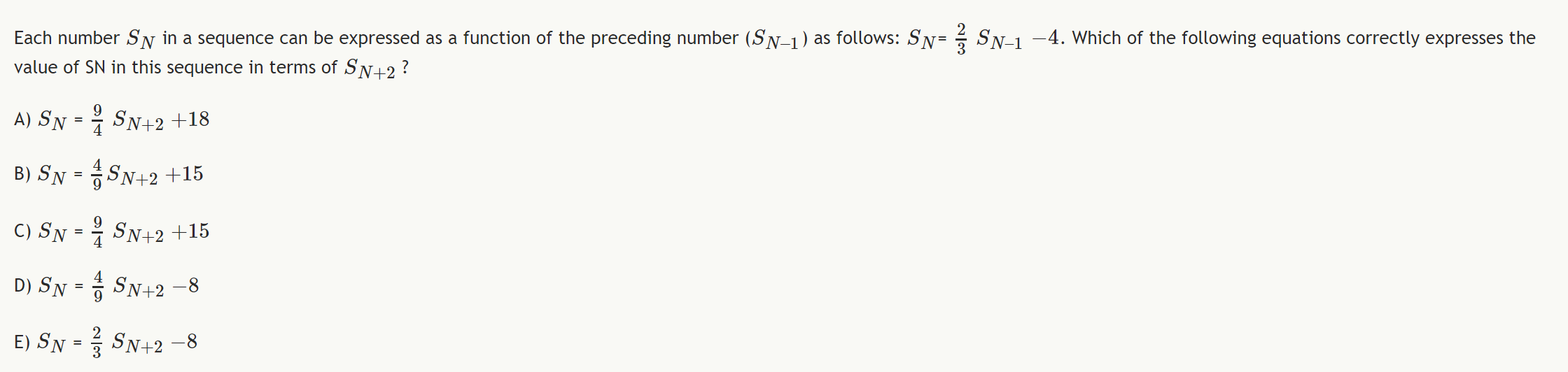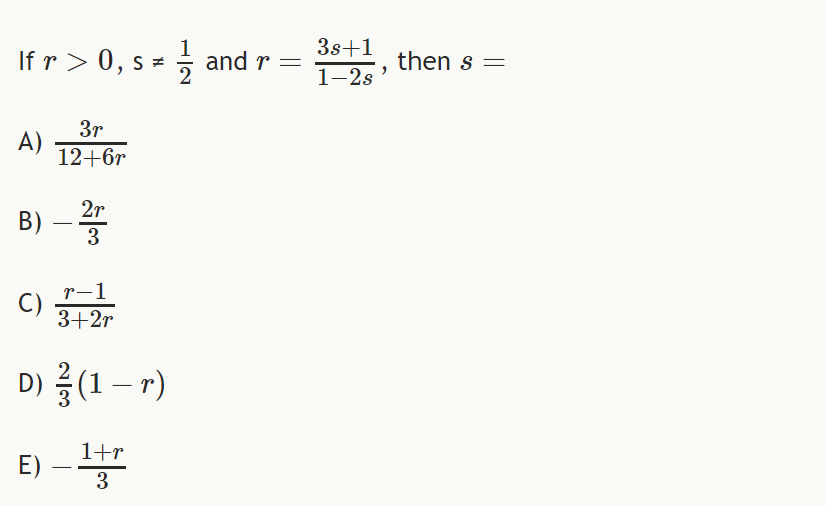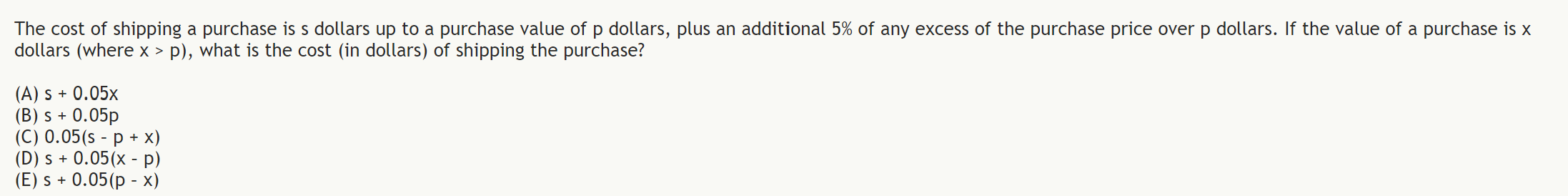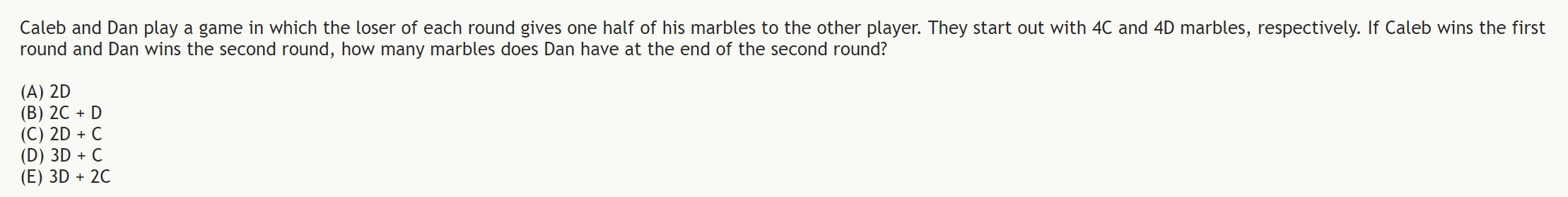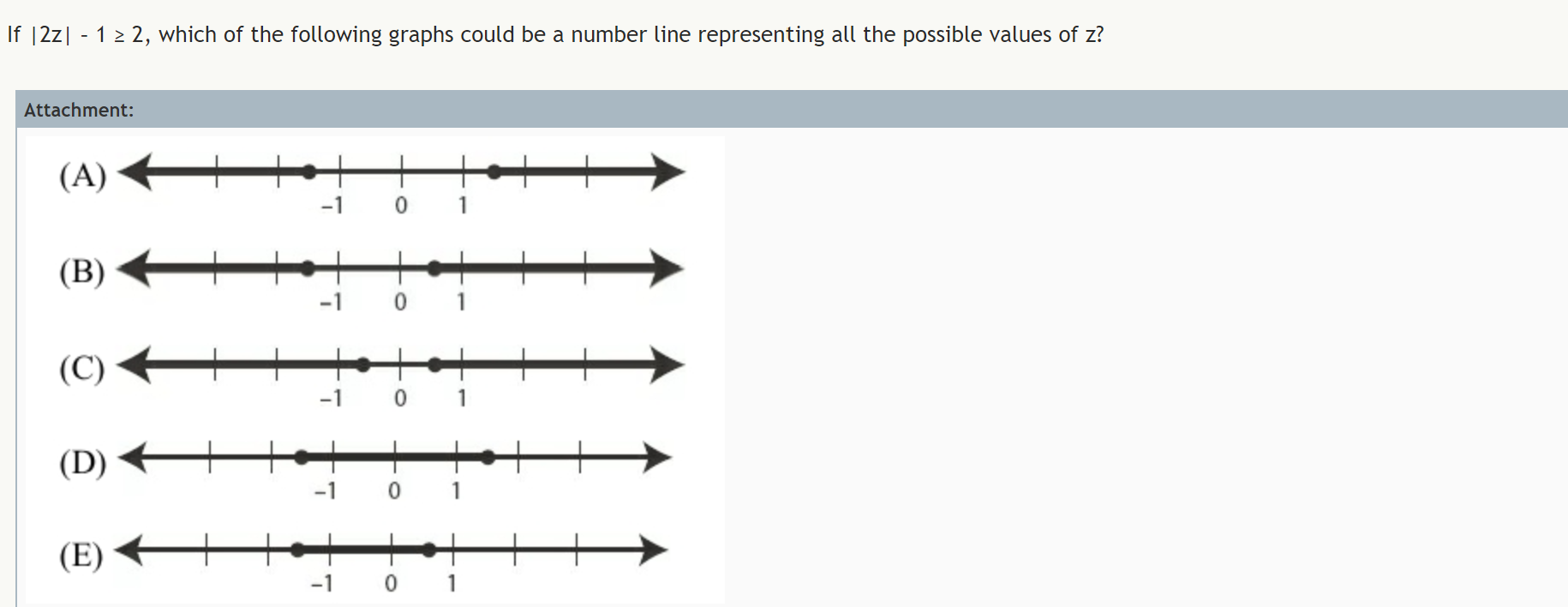Hard SAT and ACT percentages problems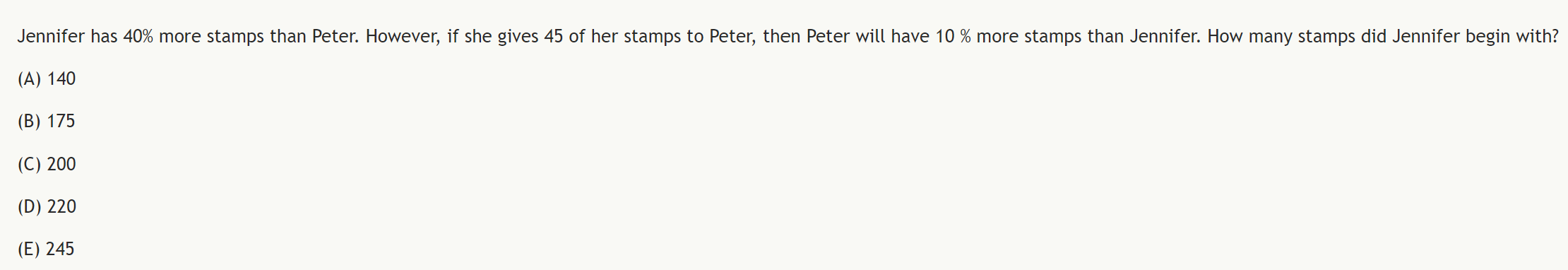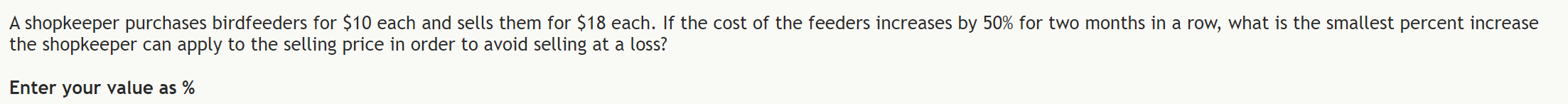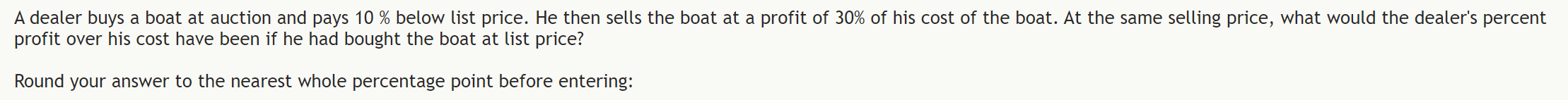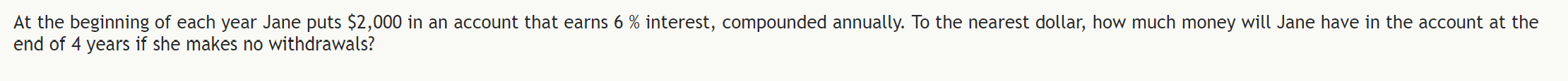Hard SAT and ACT geometry problems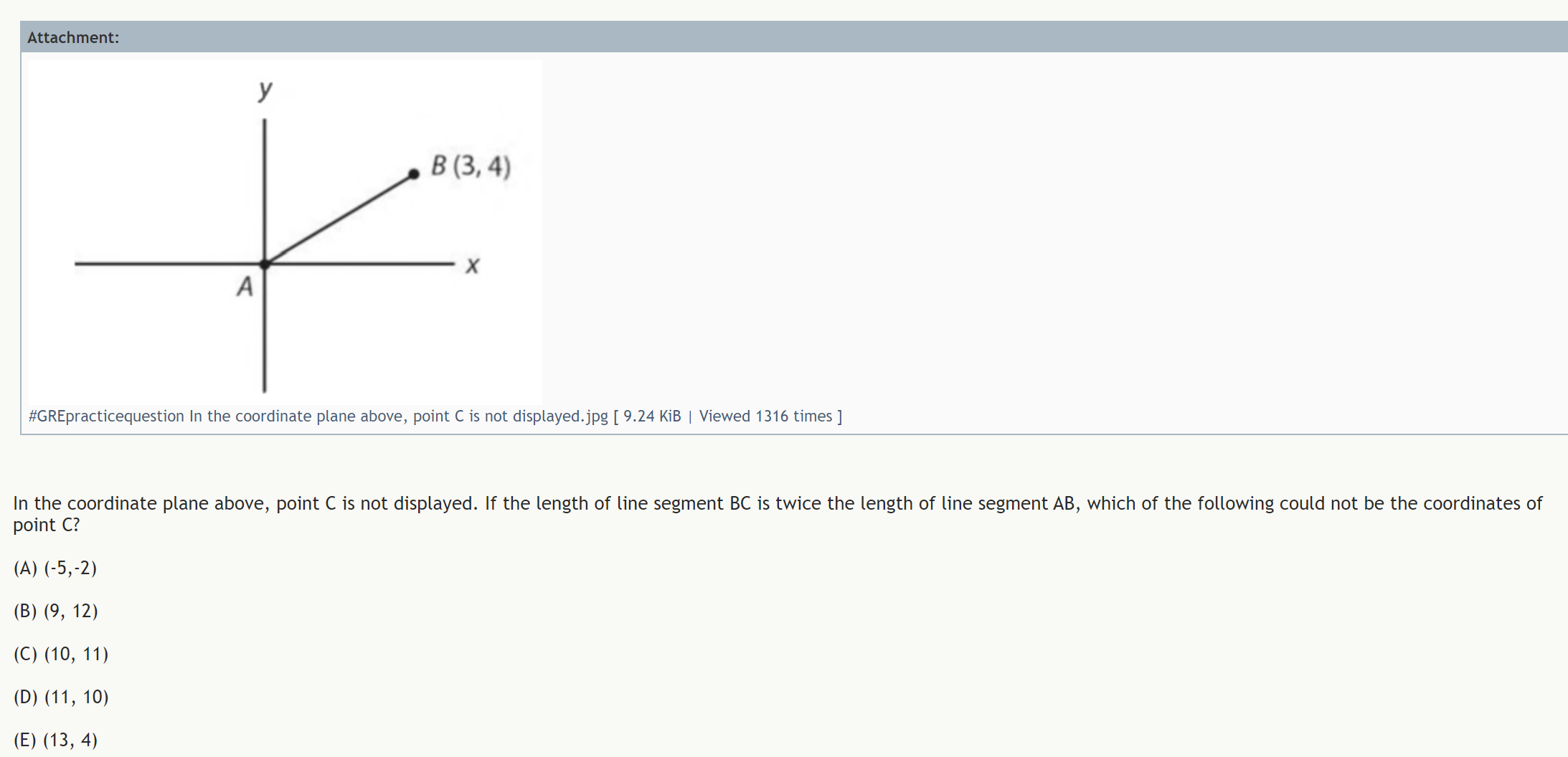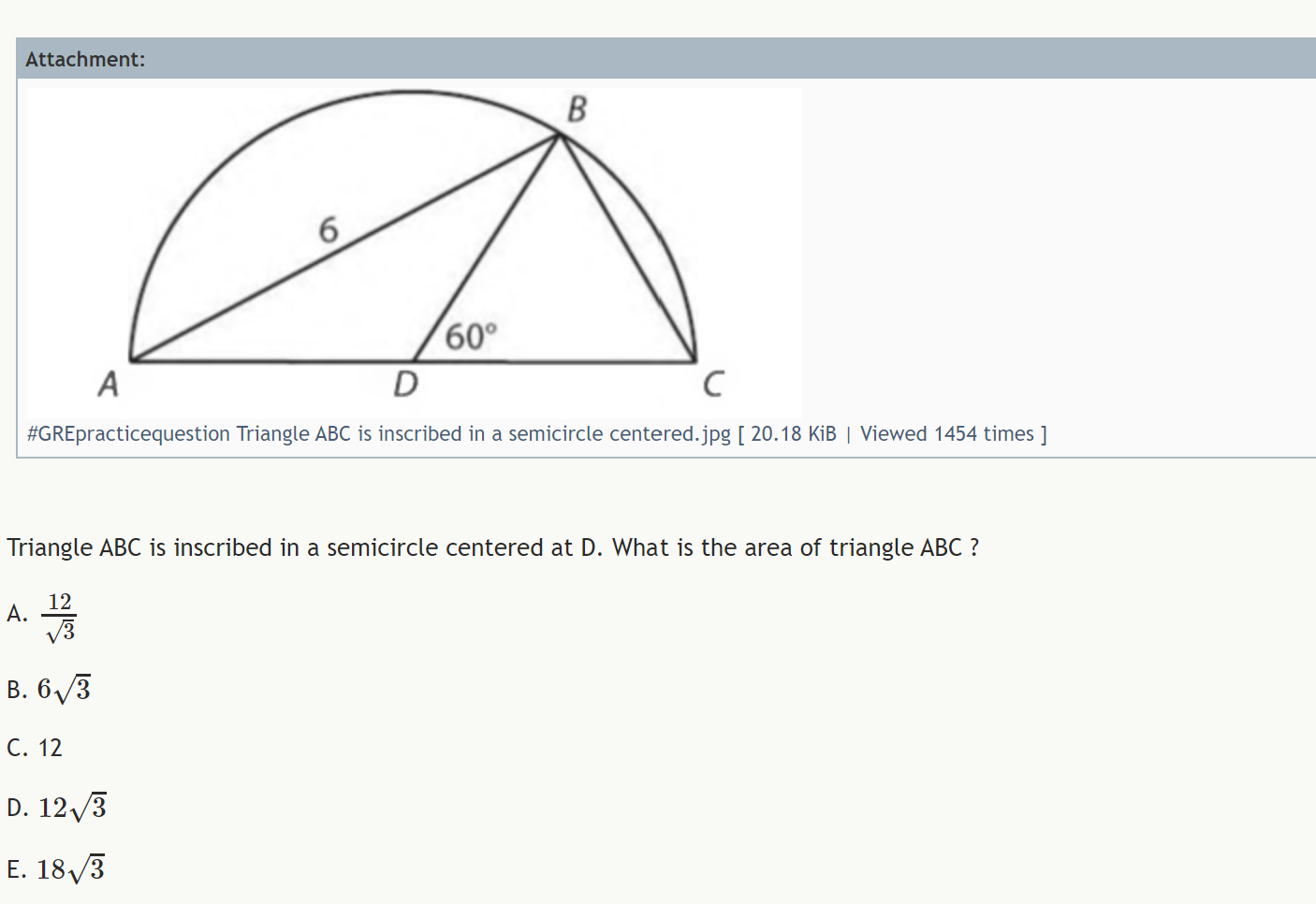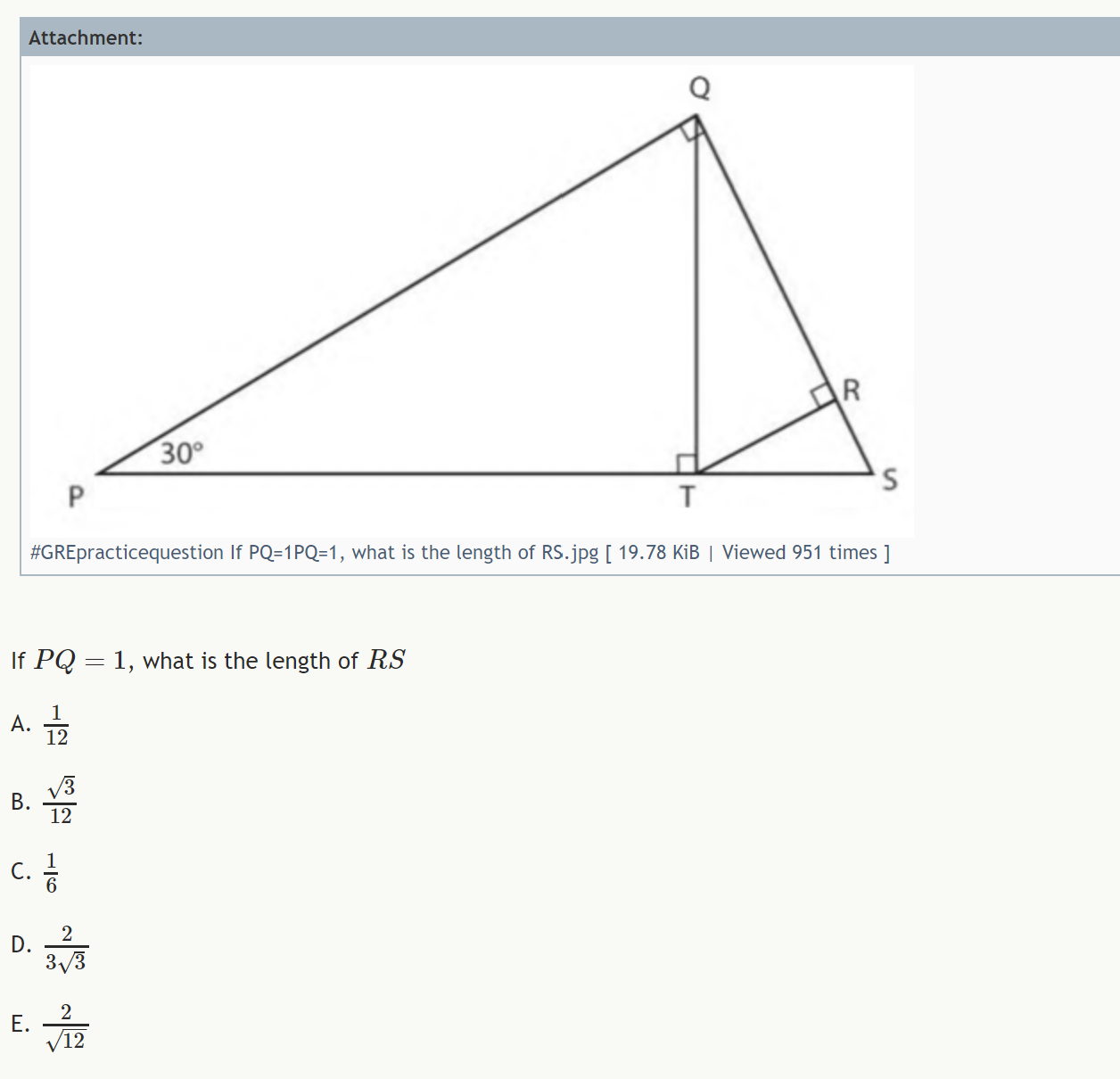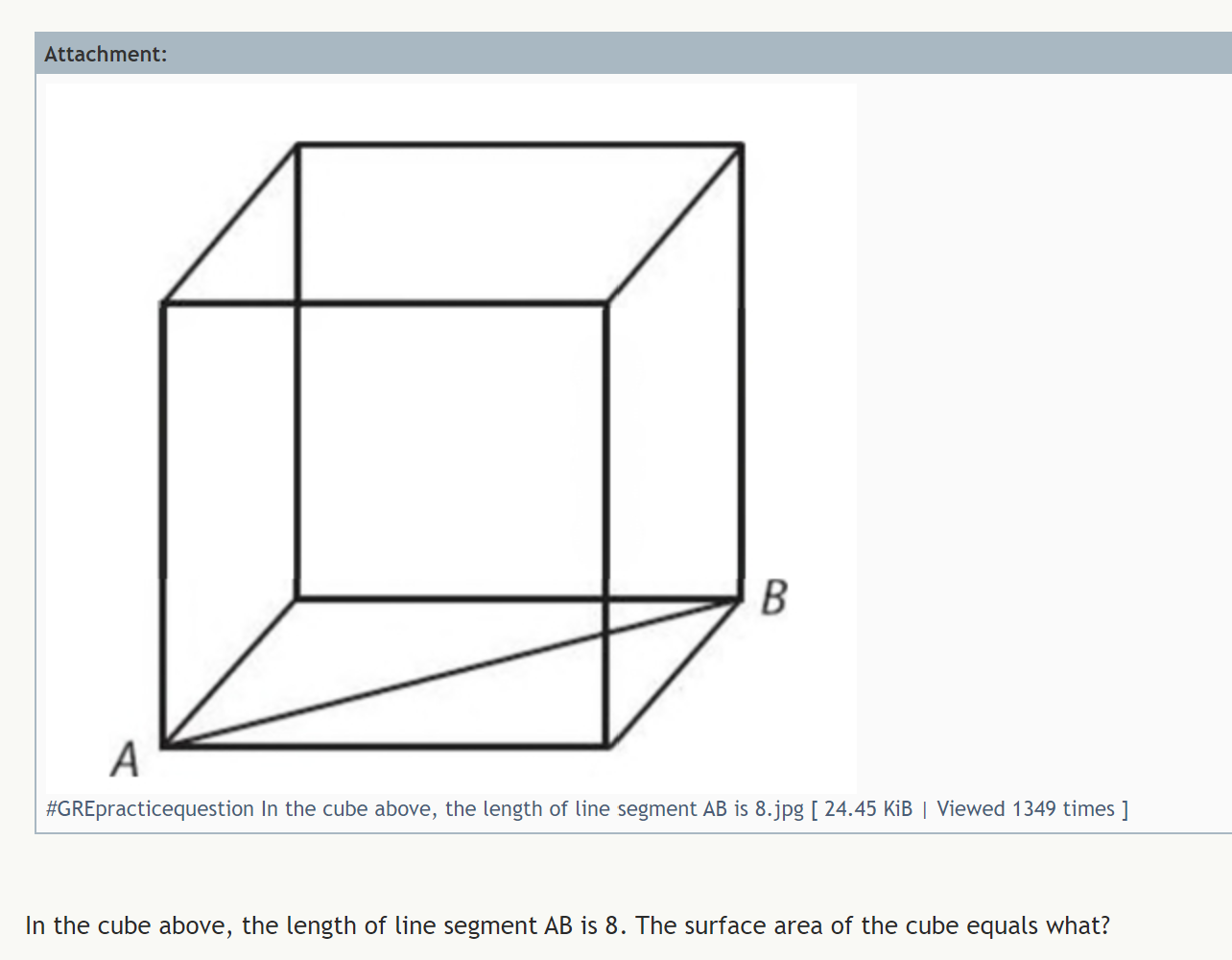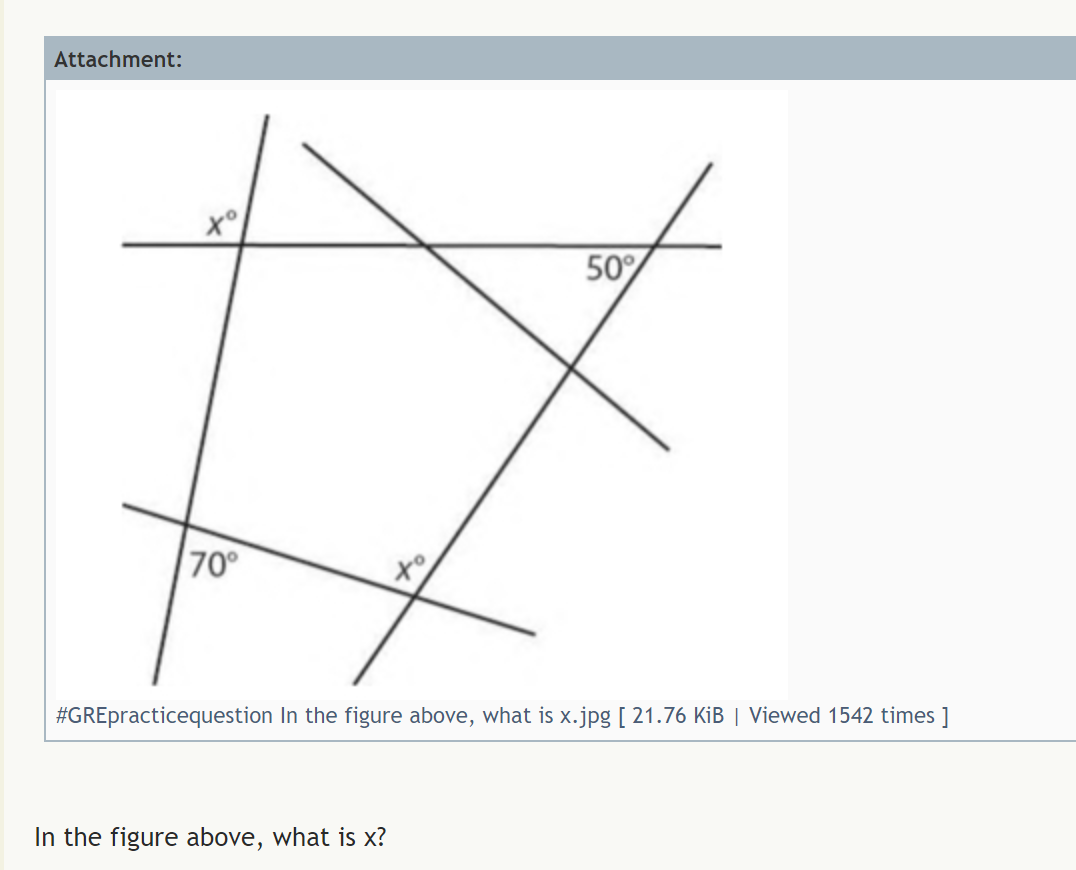Hard SAT and ACT word problems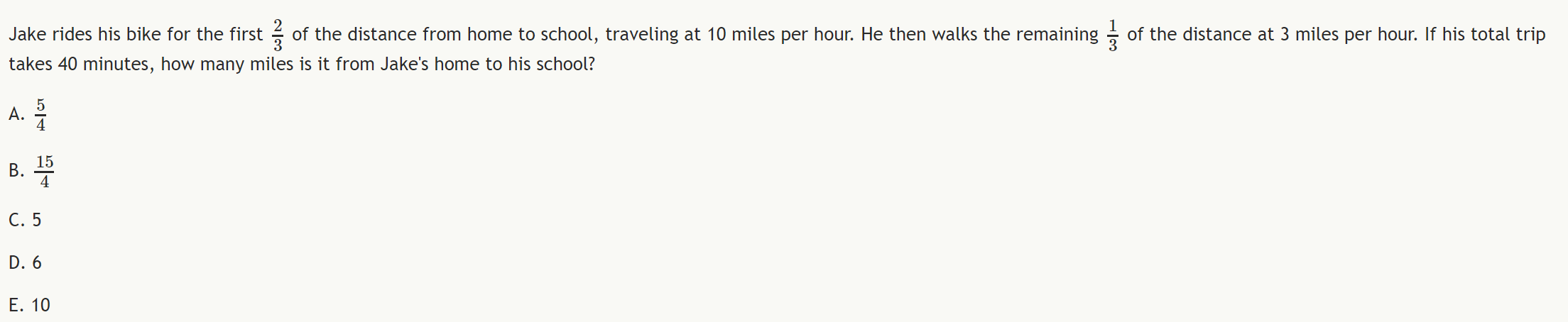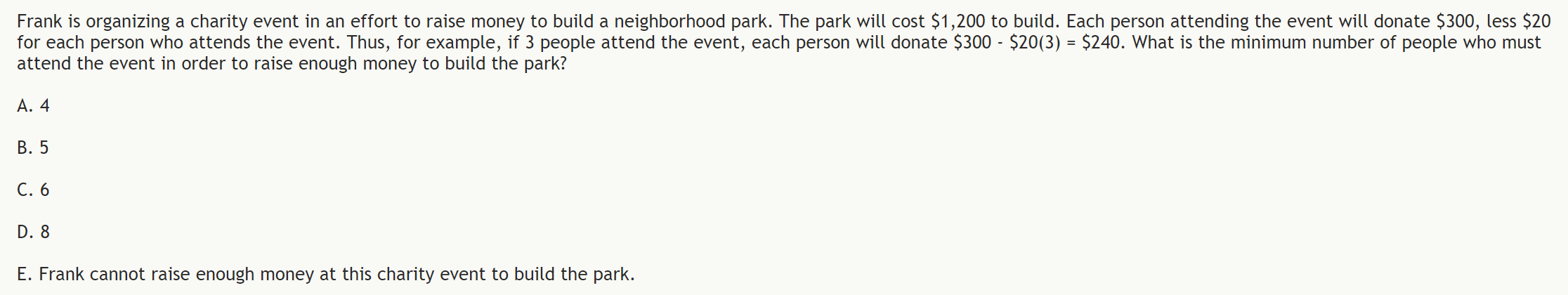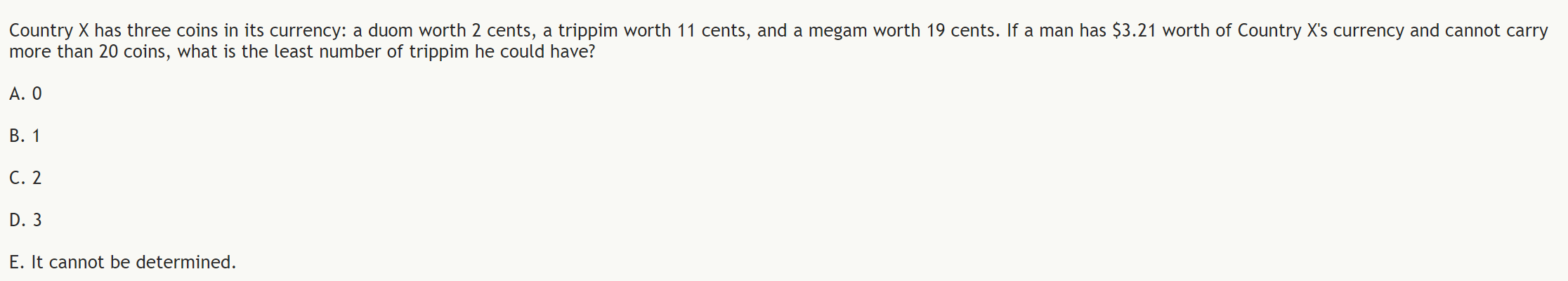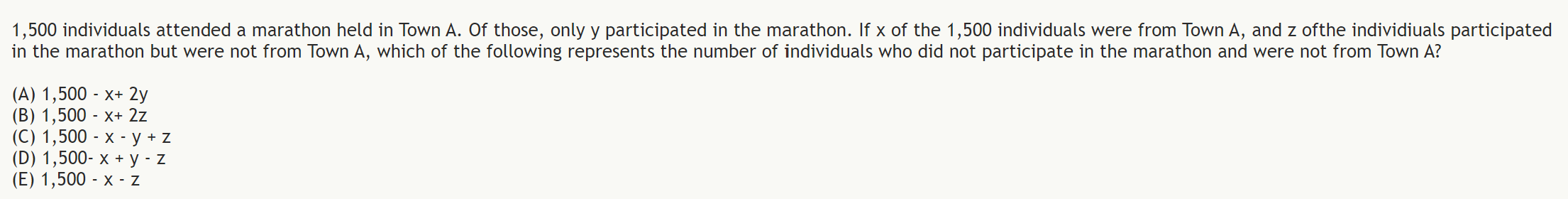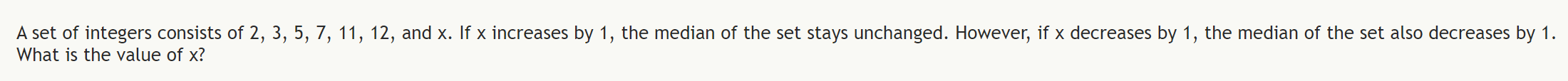Hard SAT and ACT functions problems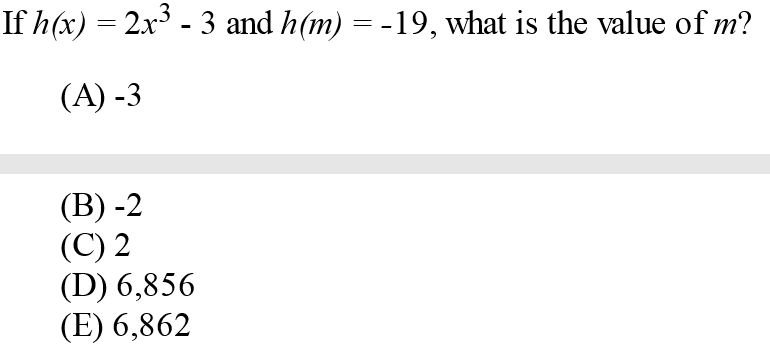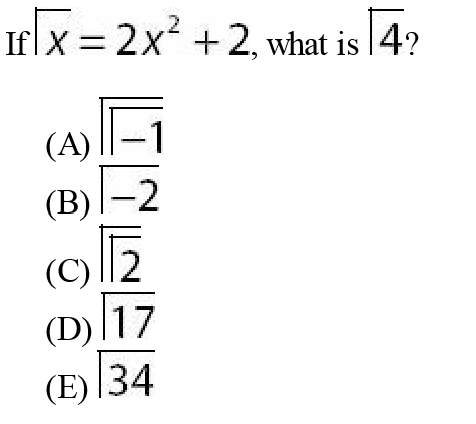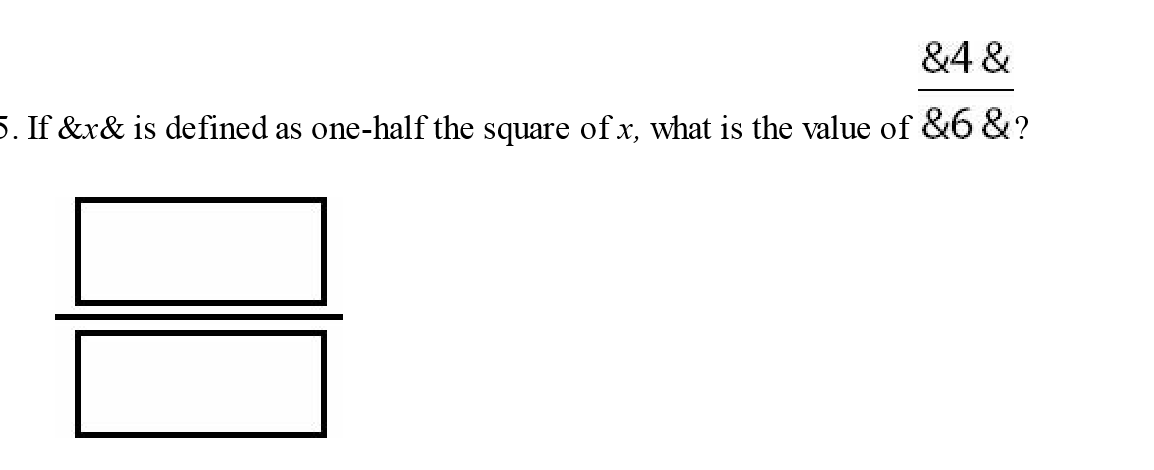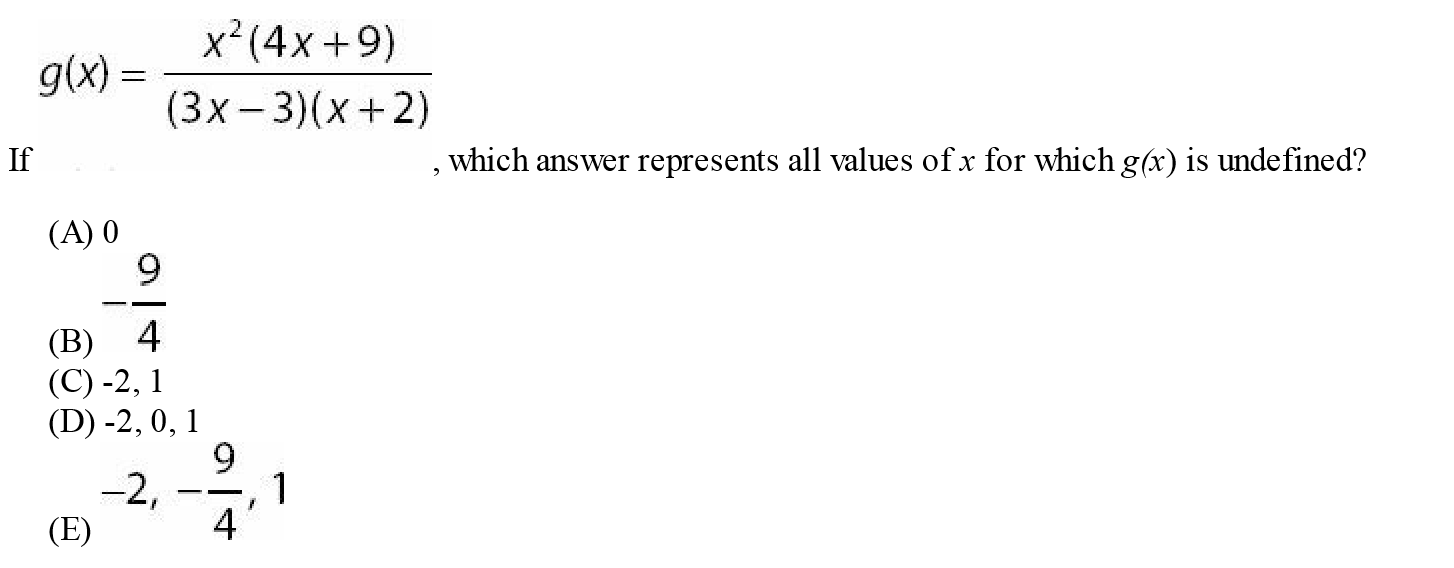Hard SAT and ACT coordinate geometry problems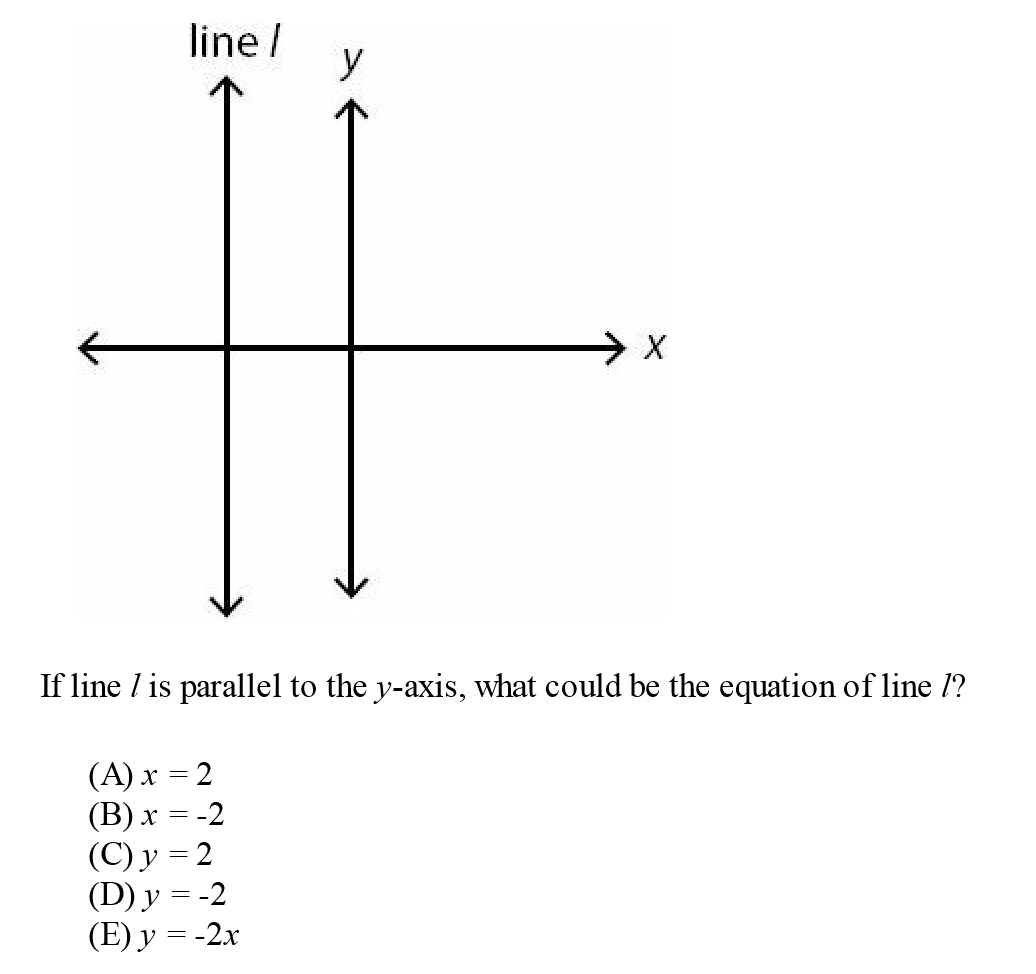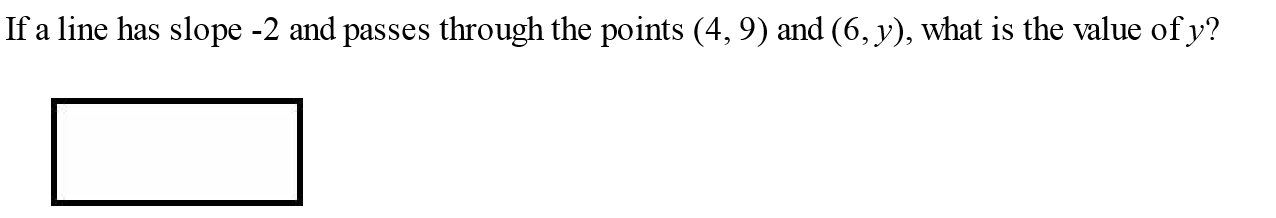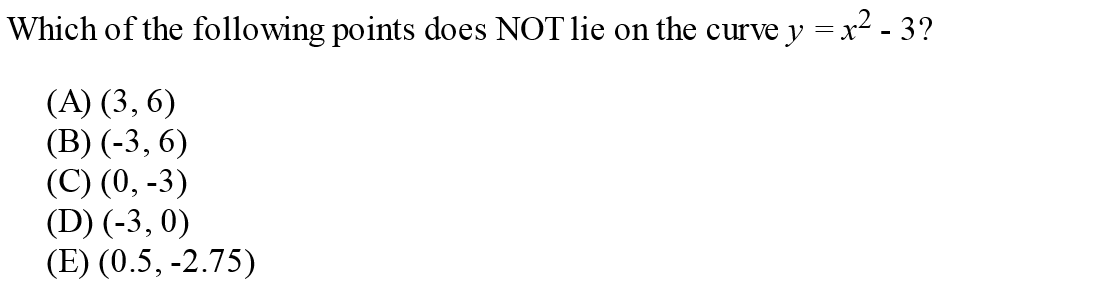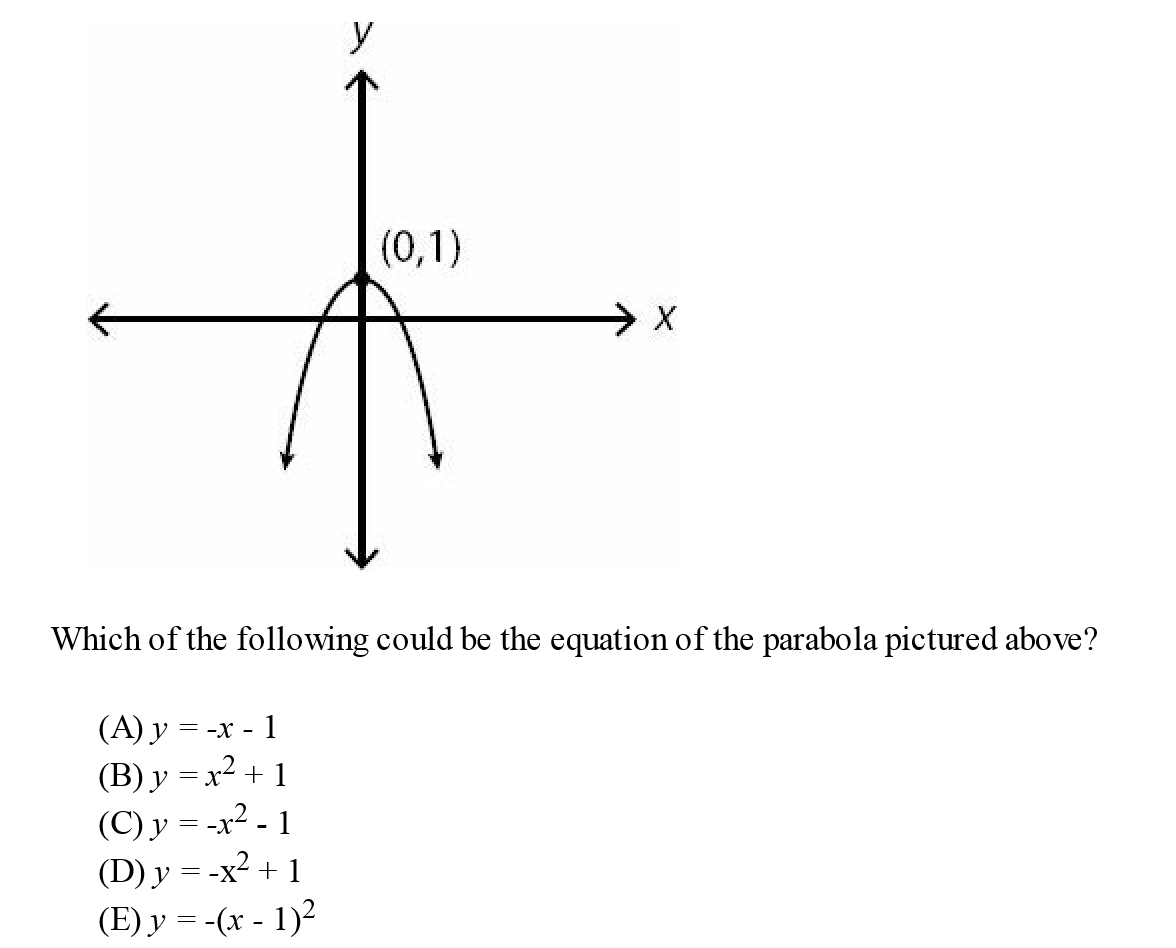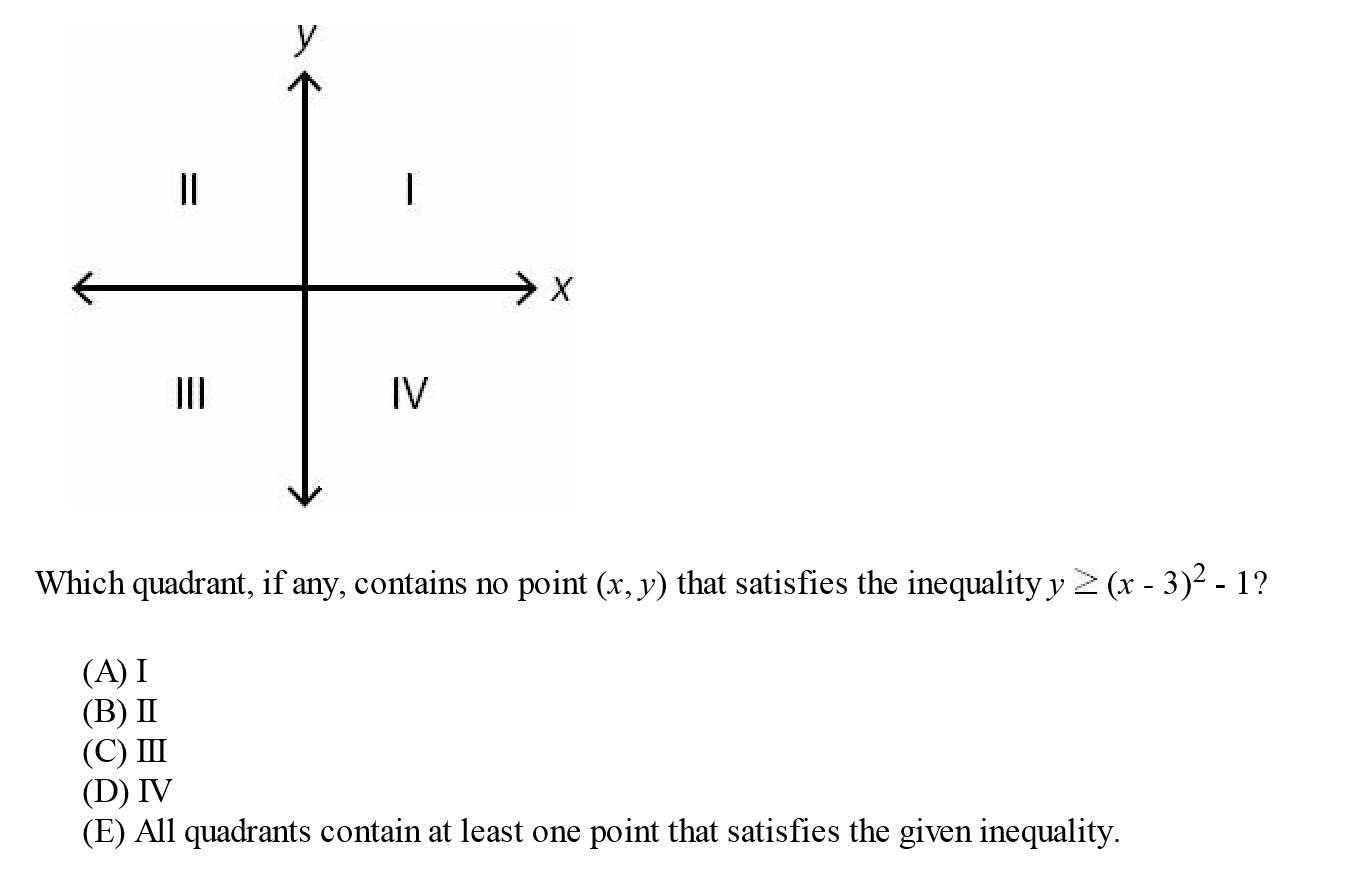Hard SAT and ACT exponents problems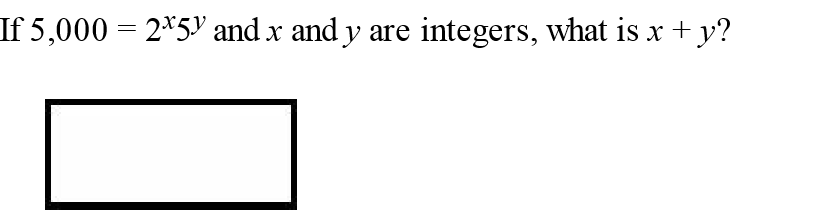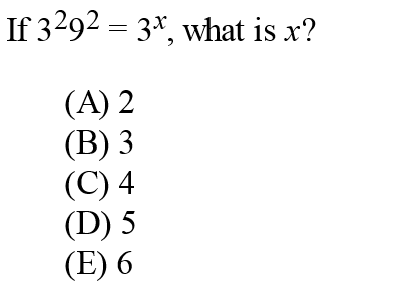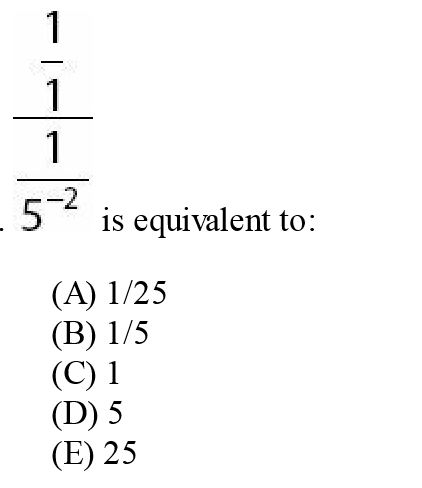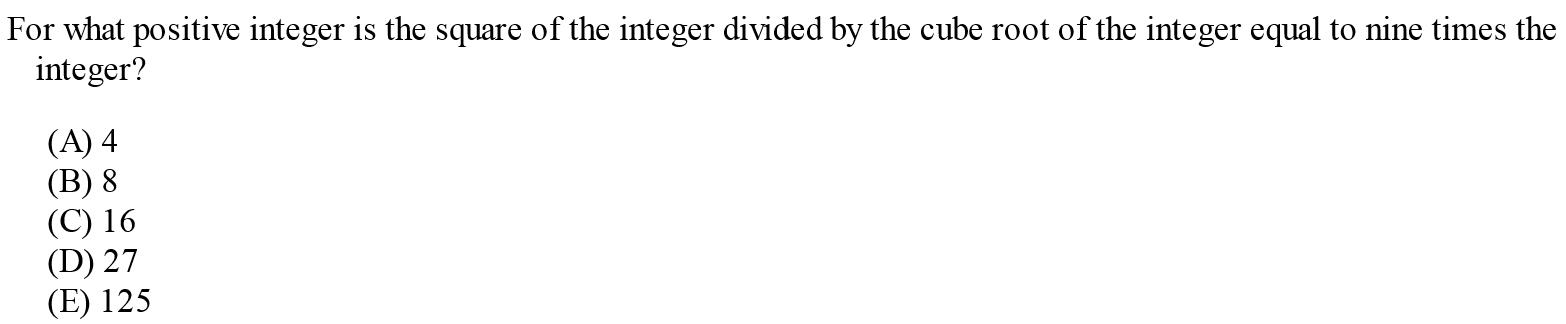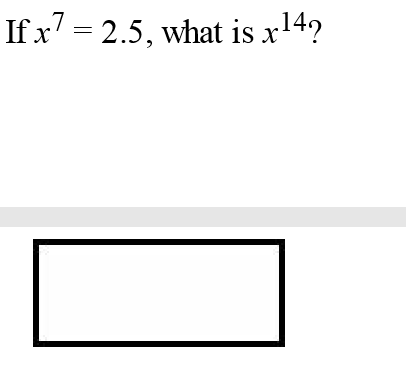Hard SAT and ACT graph problems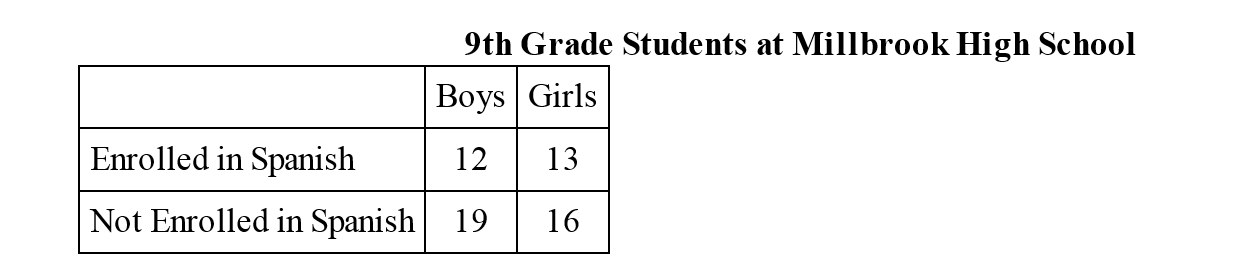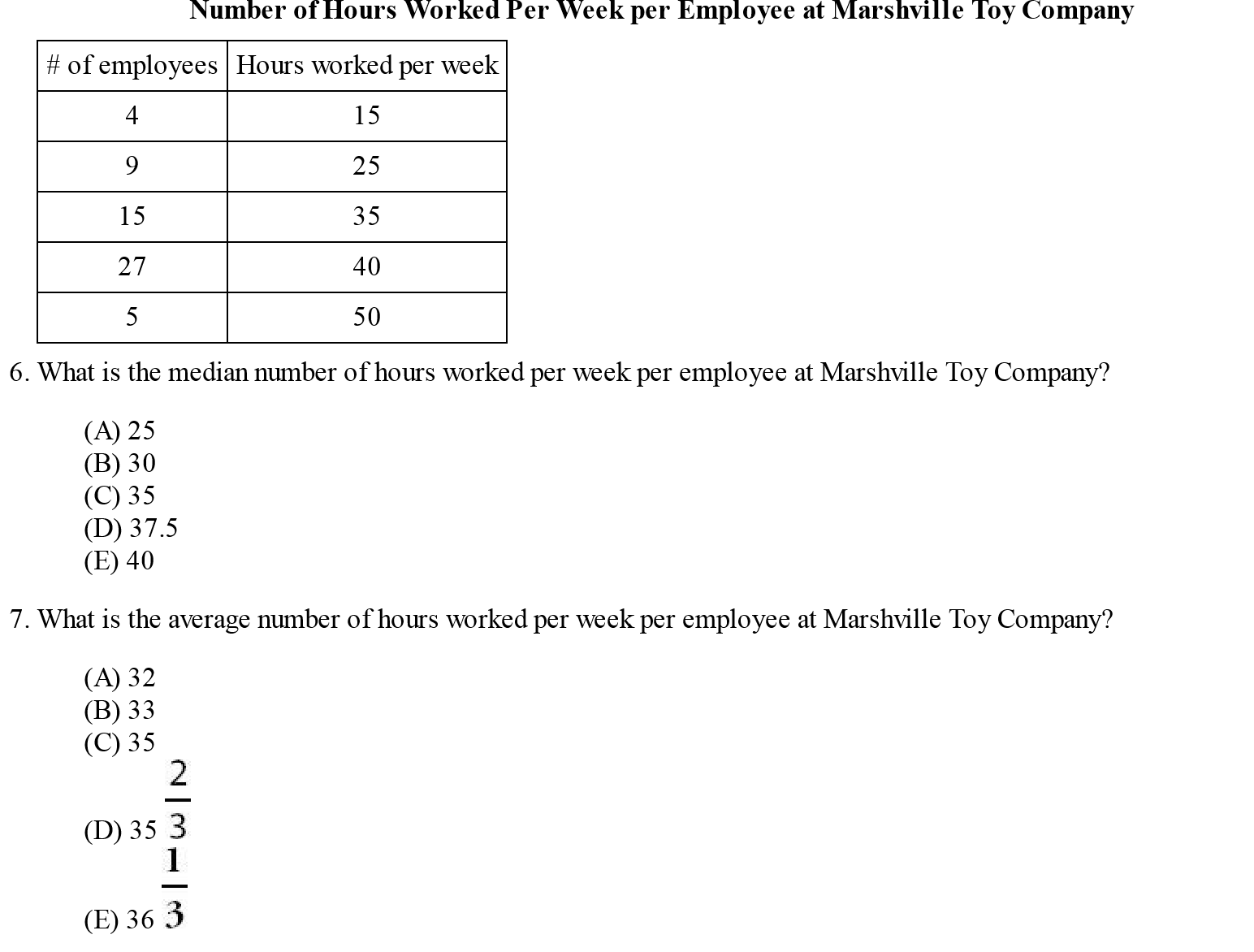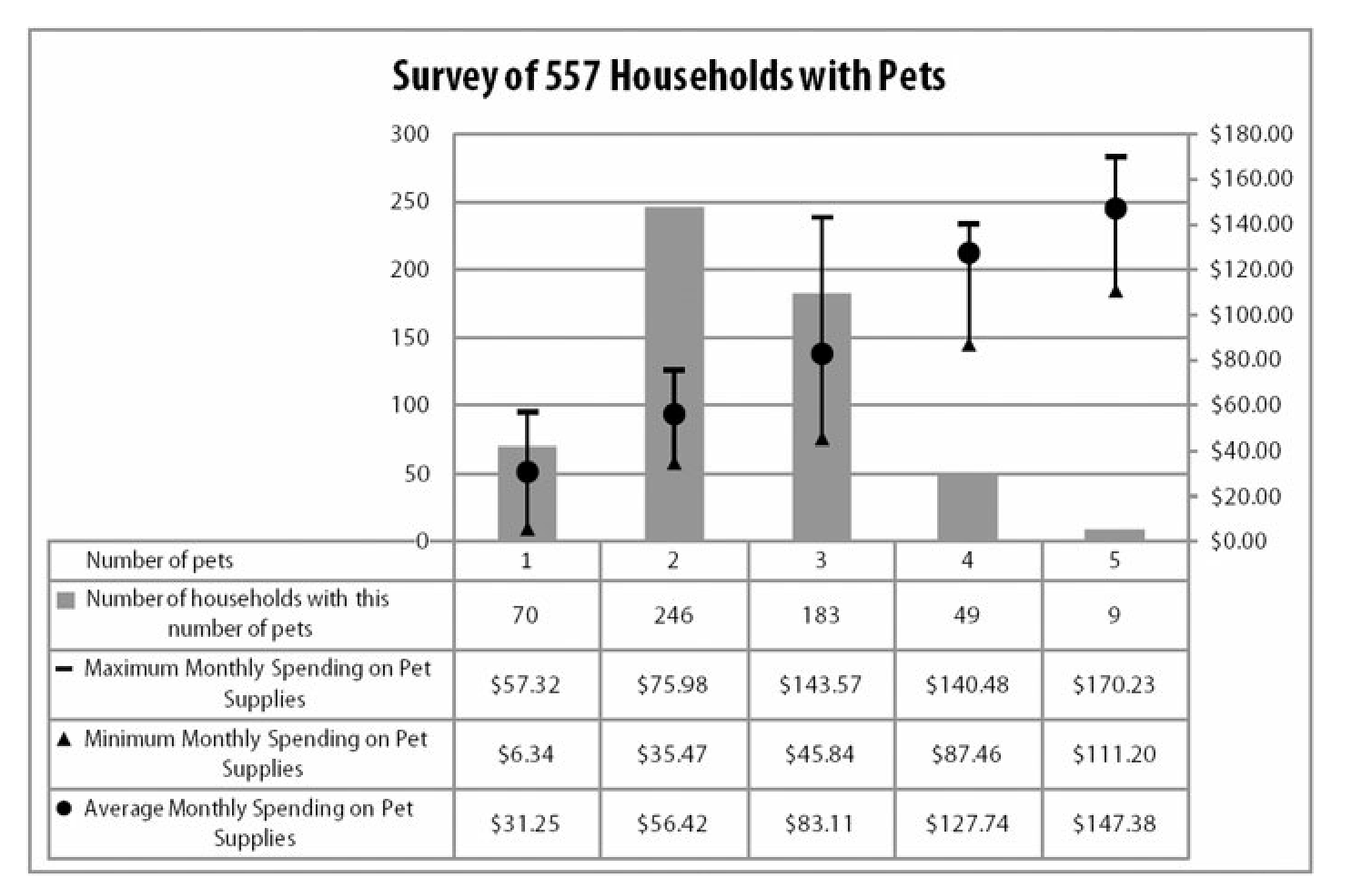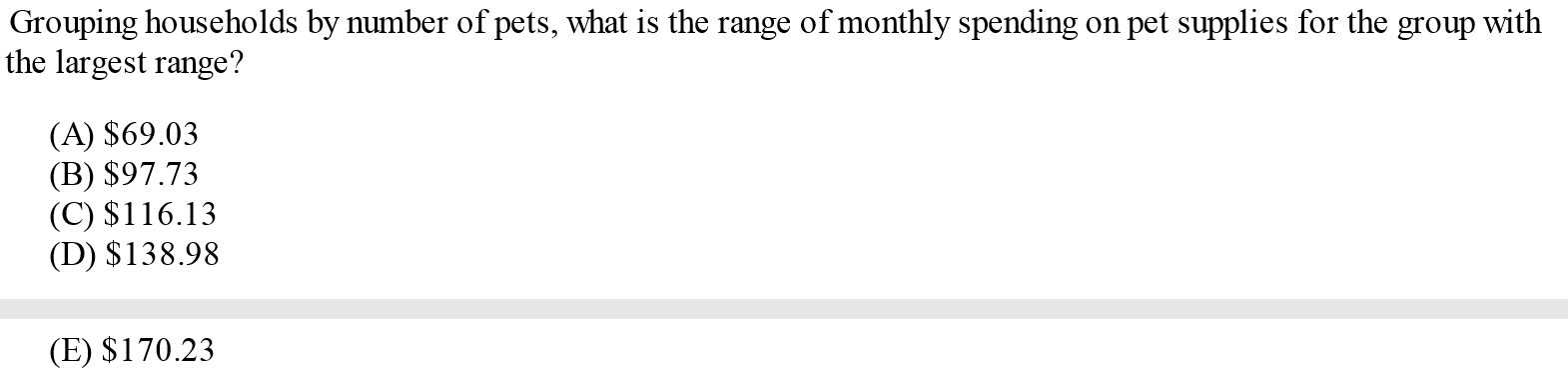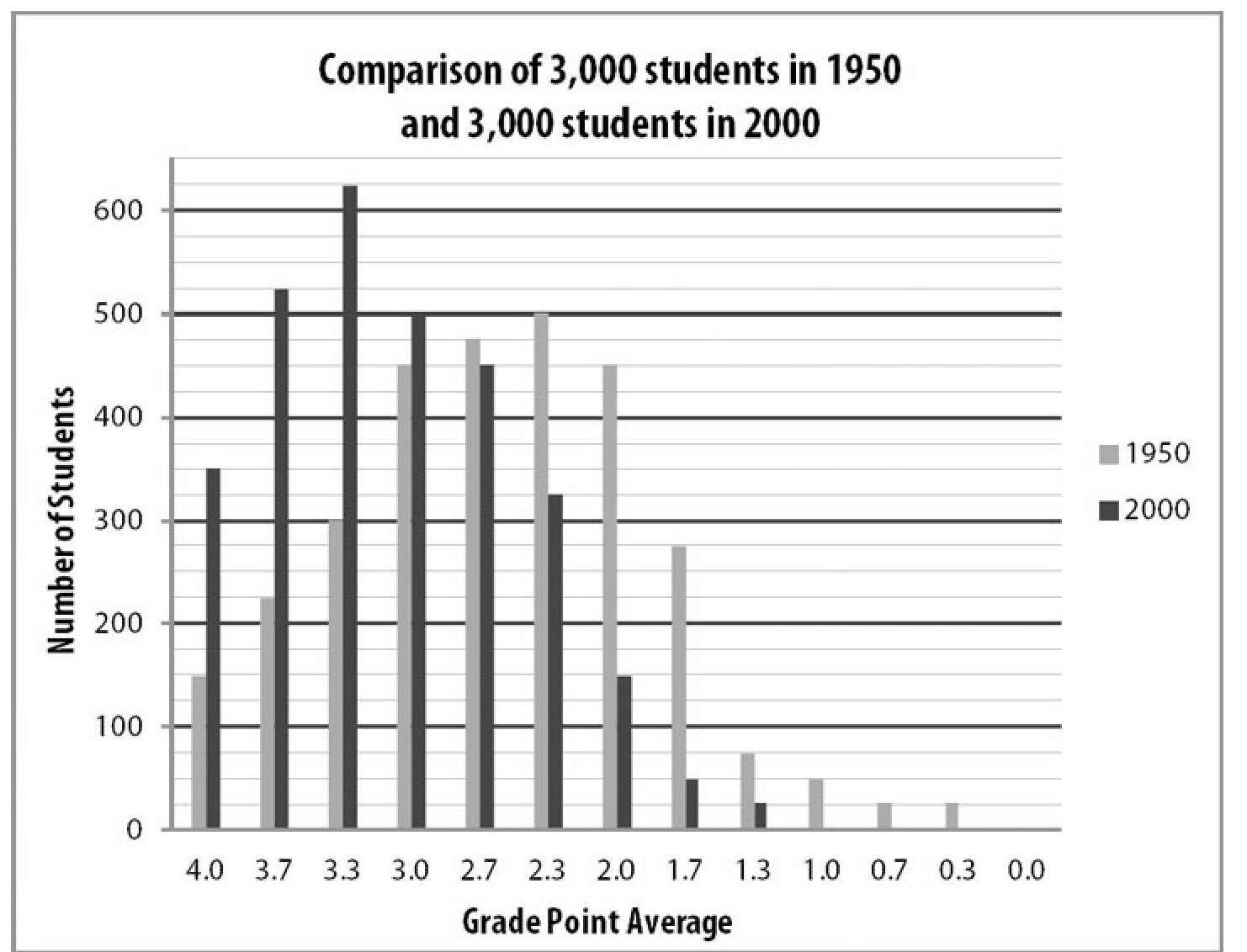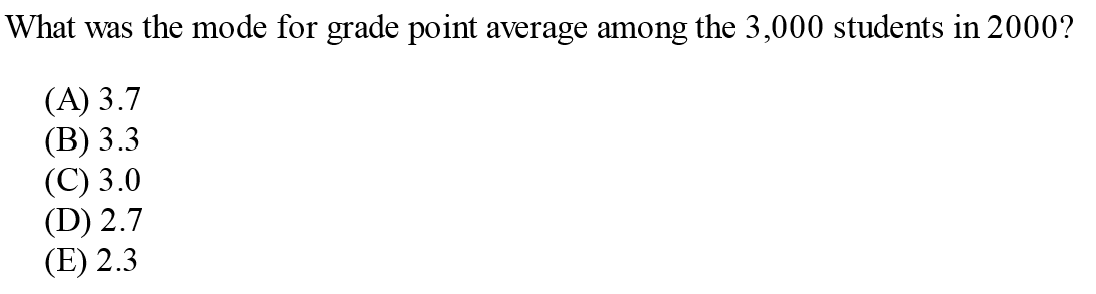Hard SAT and ACT averages questions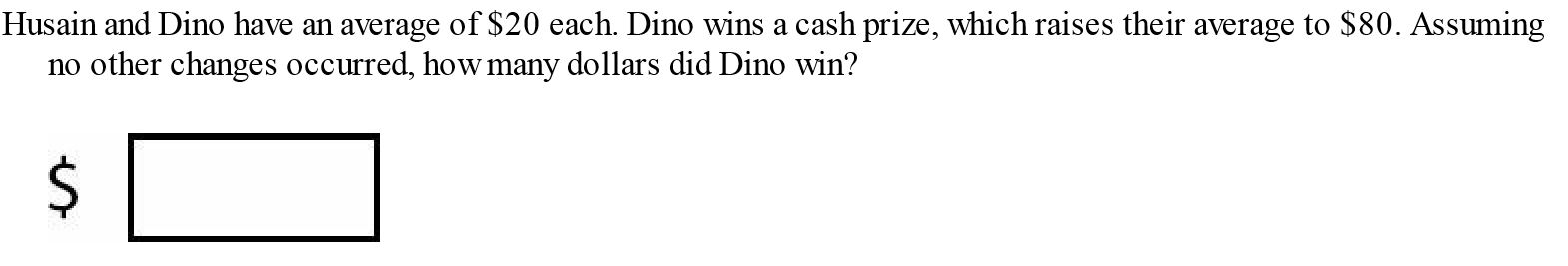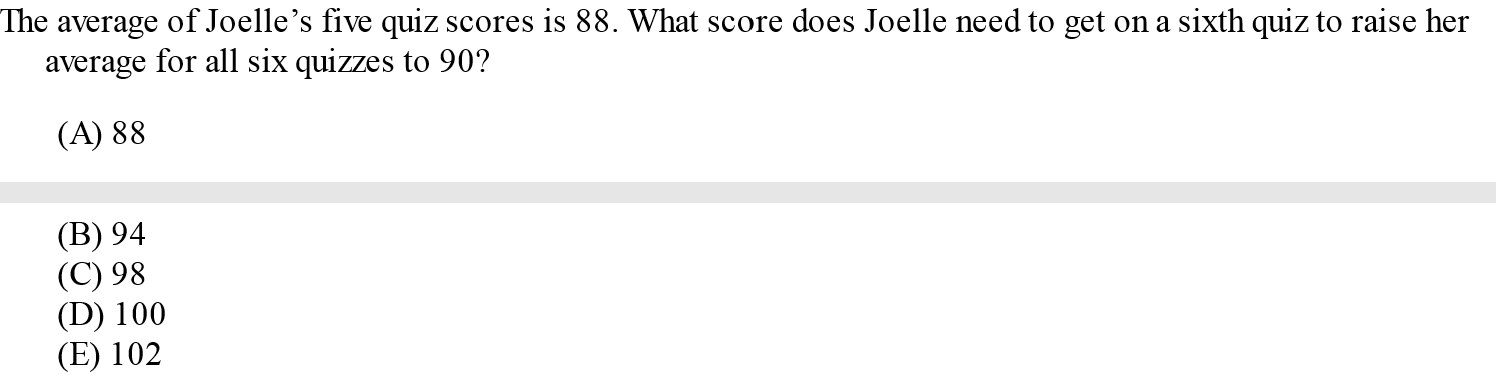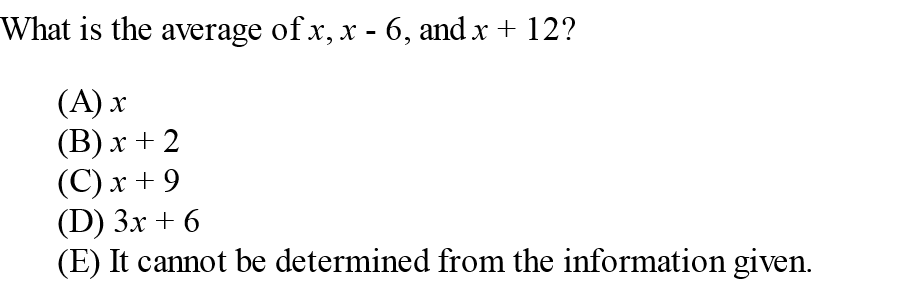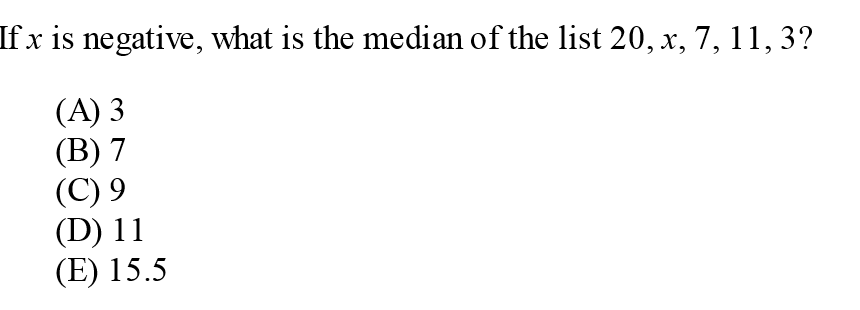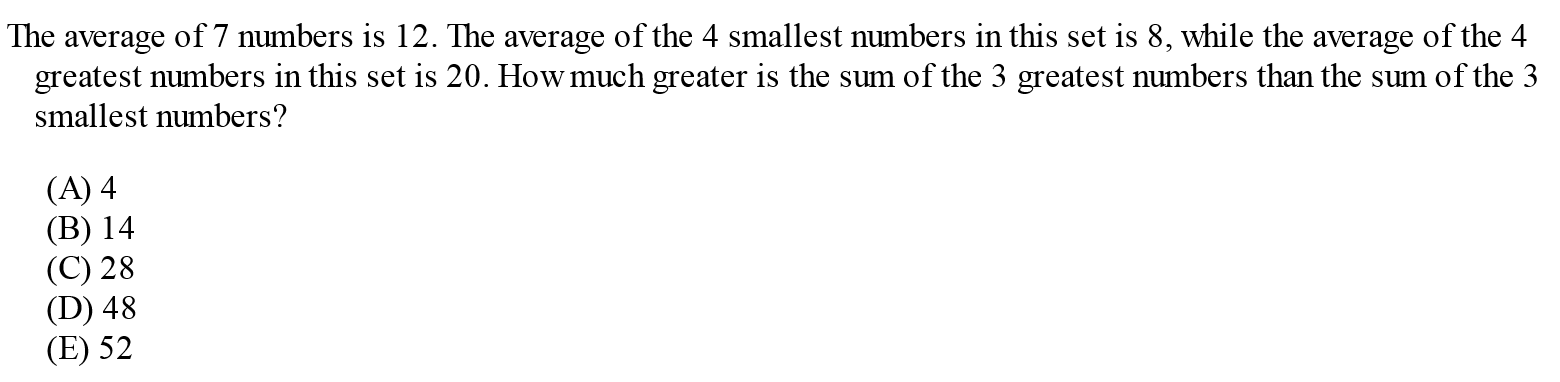1. C
2. C
3. D
4. E
5. A

1. E

2. 25

3. 17

4. 9274

1. C

2. B

3. B

4. 192

5. 100

1. B
2. E
3. B
4. E
5. 7

1. D

2. B

3. A

4. 4/9

5. C

1. B
2. 5
3. D
4. D
5. C

1. 7

2. E

3. E

4. D

5. 6.25

1. E
2. E
3. D
4. B
5. B

1. \$120

2. D

3. B

4. B

5. D